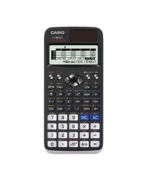Getting Started fx-991EX Archives - Casio Calculators

# Getting Started fx-991EX

Displaying Resources

FilterHow to Video
A-Level GCSE
Table of Values fx-991EX

How to create a table of values of a function with incremental steps. Extend th...How to Video
Memory Functions fx-991EX

How to store results and calculated values. Perform operations with recalled val...How to Video
A-Level GCSE
Grouped Data Statistics

How to enter grouped data in a frequency table and calculate the mean, quartiles...How to Video
A-Level
Calculus

How to integrate a function between limits to find the definite integral. Differ...How to Leaflet
fx-991EX A-Level GCSE
Statistics Quick Start fx-991EX

How to calculate single variable and bivariate statistics such as mean, standard...How to Leaflet
fx-991EX A-Level GCSE
Table Quick Start fx-991EX

How to calculate a table of values for a function. Change the step interval and ...Reference Leaflet
fx-991EX
fx-991EX Quick Start Guide

Overview of the menu functions of the fx-991EX with examples for the key apps. C...Reference Leaflet
fx-991EX
Function Keys Quick Start fx-991EX

An overview of the functions available on the fx-991EX and the individual featur...How to Leaflet
fx-991EX A-Level GCSE
Equation Quick Start fx-991EX

How to solve simultaneous equations with up to 4 unknowns. Solve polynomial equa...How to Leaflet
fx-991EX A-Level
Distribution Quick Start fx-991EX

How to calculate probabilities for binomial, normal and poisson distributions. C...How to Leaflet
fx-991EX A-Level GCSE
Calculate – Maths Quick Start

How to enter basic calculations on the fx-991EX. Includes logarithms summation, ...